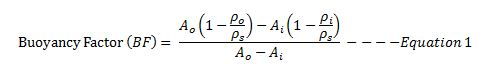## Buoyancy Factor with Two Different Fluid Weights in The WellBuoyancy Factor is the factor that is used to compensate loss of weight due to immersion in drilling fluid and you can find more information from this article > buoyancy factor calculation .  In that article, it demonstrates the buoyancy formula only for one fluid in the wellbore. However, this time, we will have the details about buoyancy factor when inside and outside fluid are different.

Buoyancy factor with different fluid inside and outside of tubular is listed below;Where;

Ao is an external area of the component.

Ai is an internal area of the component.

ρo is fluid density in the annulus at the component depth in the wellbore.

ρi is fluid density in the component depth in the wellbore.

ρs is steel weight density. Steel density is 65.4 ppg.

Buoyancy factor is the factor that is used to compensate loss of weight due to immersion in drilling fluid and you can find the calculation from here http://www.drillingformulas.com/buoyancy-factor-calculation/.We have created a simple table to help people determine the buoyancy factor quickly. Let’s take a look at the table. In Figure 1, it shows the main page and you can select the mud weight range from 4.0 ppg to 19.0 ppg.Figure 1– Main Page BF Table

For instant, we choose 8.0 ppg and the table will show buoyancy from 8.0 – 8.9 ppg (Figure 2)Figure 2 – Buoyancy Factor for 8 ppg Range

## Buoyancy Factor CalculationBuoyancy Factor is the factor that is used to compensate loss of weight due to immersion in drilling fluid.

Before explaining any further, I will explain you about the basic concept of Buoyancy. Buoyancy is the upward force that keeps things afloat. The net upward buoyancy force equals to the amount of the weight of fluid displaced by the body volume. This force will make objects lighter when it immerses in fluid. For example, we feel ourselves lighter when we are in swimming pool because this is the effect of buoyancy.In drilling operation, we need to know how much weight of string of drill pipe, completion string, etc in drilling fluid. Therefore,  Buoyancy Factor is value that we need to know and be able to calculate this value. Please follow the formulas below to calculate Buoyancy Factor in different mud weight units, ppg and lb/ft3.

## Buoyancy Factor using mud weight in ppg

Buoyancy Factor (BF) = (65.5 – mud weight in ppg) ÷65.5

Note: 65.5 ppg is density of steel.

Example: Determine the buoyancy factor for a 13.0 ppg fluid:
BF = (65.5 – 13.0) ÷ 65.5
BF = 0.8015

## Buoyancy Factor using mud weight in  lb/ft3

Buoyancy Factor (BF) = (489 – mud weight in lb/ft3) ÷489

Note: 489 lb/ft3 is density of steel.

Example: Determine the buoyancy factor for a 100 lb/ft3 fluid:
BF = (489 – 100) ÷489
BF = 0.7955

## How to use the Buoyancy Factor

In order to figure out the actual weight of drilling string in mud, the air weight of drilling string times the buoyancy factor equal to actual weight in mud, called buoyed weight.

Example: Determine the string weight in 13.0 ppg mud. Air weight of string is 350 klb.

The buoyancy factor for a 13.0 ppg fluid:
BF = (65.5 – 13.0) ÷ 65.5
BF = 0.8015

Buoyed weight of drill string = String weight in the air x BF

The buoyed weight of drill string in 13.0 ppg mud = 350 x 0.8015 = 280.5 Klb.

Ref books: Lapeyrouse, N.J., 2002. Formulas and calculations for drilling, production and workover, Boston: Gulf Professional publishing.

Bourgoyne, A.J.T., Chenevert , M.E. & Millheim, K.K., 1986. SPE Textbook Series, Volume 2: Applied Drilling Engineering, Society of Petroleum Engineers.

Mitchell, R.F., Miska, S. & Aadny, B.S., 2011. Fundamentals of drilling engineering, Richardson, TX: Society of Petroleum Engineers.